# Select Odd & Even Rows & Columns from Data Frame in R (4 Examples)

In this article, I’ll explain how to extract odd and even rows and columns from a data frame in the R programming language.

Let’s jump right to the examples…

## Creating Example Data

The first step is to create some data that we can use in the following example code:

```data <- as.data.frame(matrix(1:35, ncol = 5))    # Create example data
data                                             # Print example data```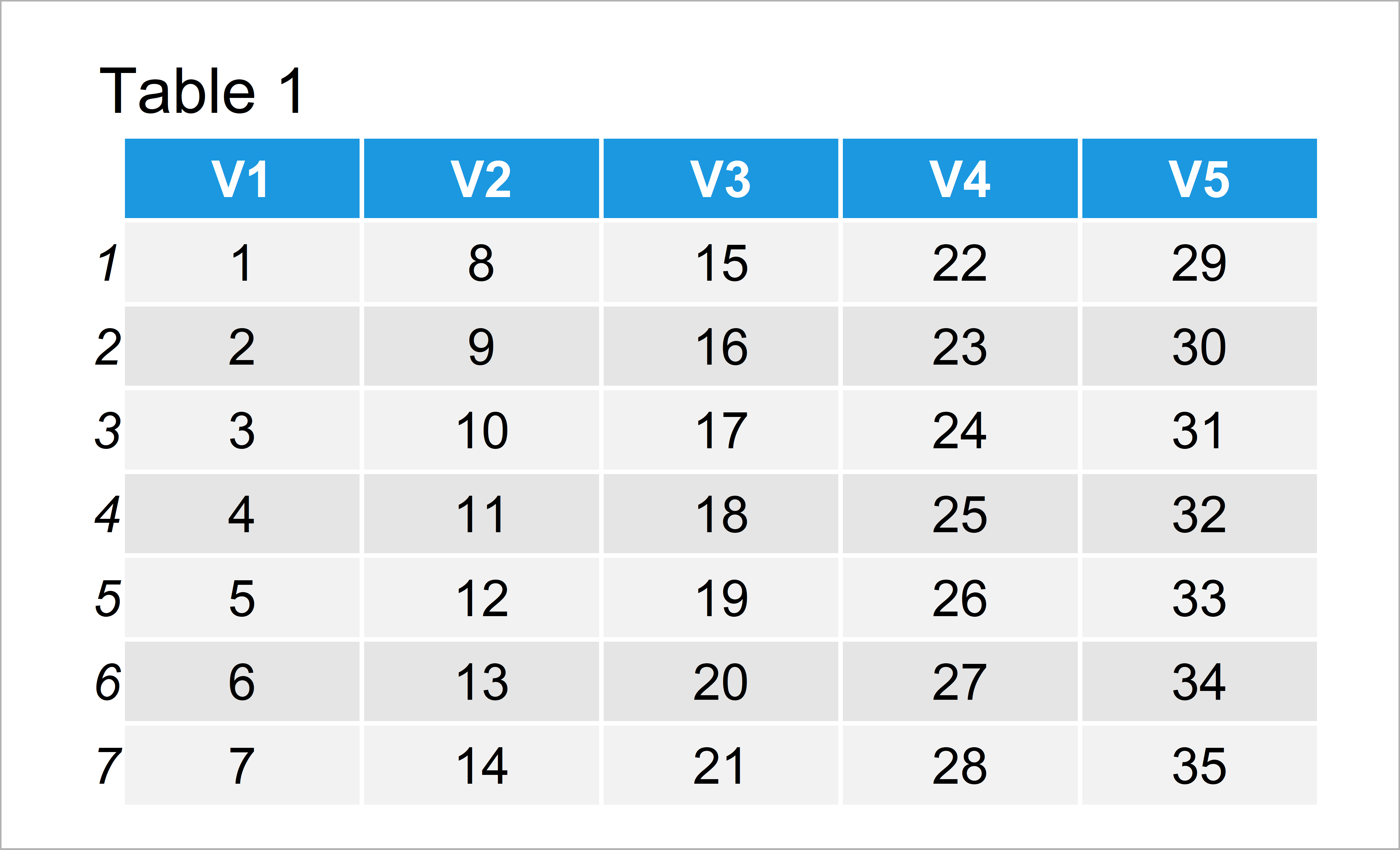Table 1 shows the structure of the example data: It is constructed of seven rows and five columns.

## Example 1: Extract Odd Rows from Data Frame

The following code explains how to subset all rows with an odd index position from a data frame object.

First, we have to create a dummy indicator that shows whether a row is even or odd.

For this, we can apply the seq_len and nrow functions as well as the %% operator.

```row_odd <- seq_len(nrow(data)) %% 2              # Create row indicator
row_odd                                          # Print row indicator
#  1 0 1 0 1 0 1```

Next, we can use our dummy to drop all even rows from our data frame:

```data_row_odd <- data[row_odd == 1, ]             # Subset odd rows
data_row_odd                                     # Print odd rows```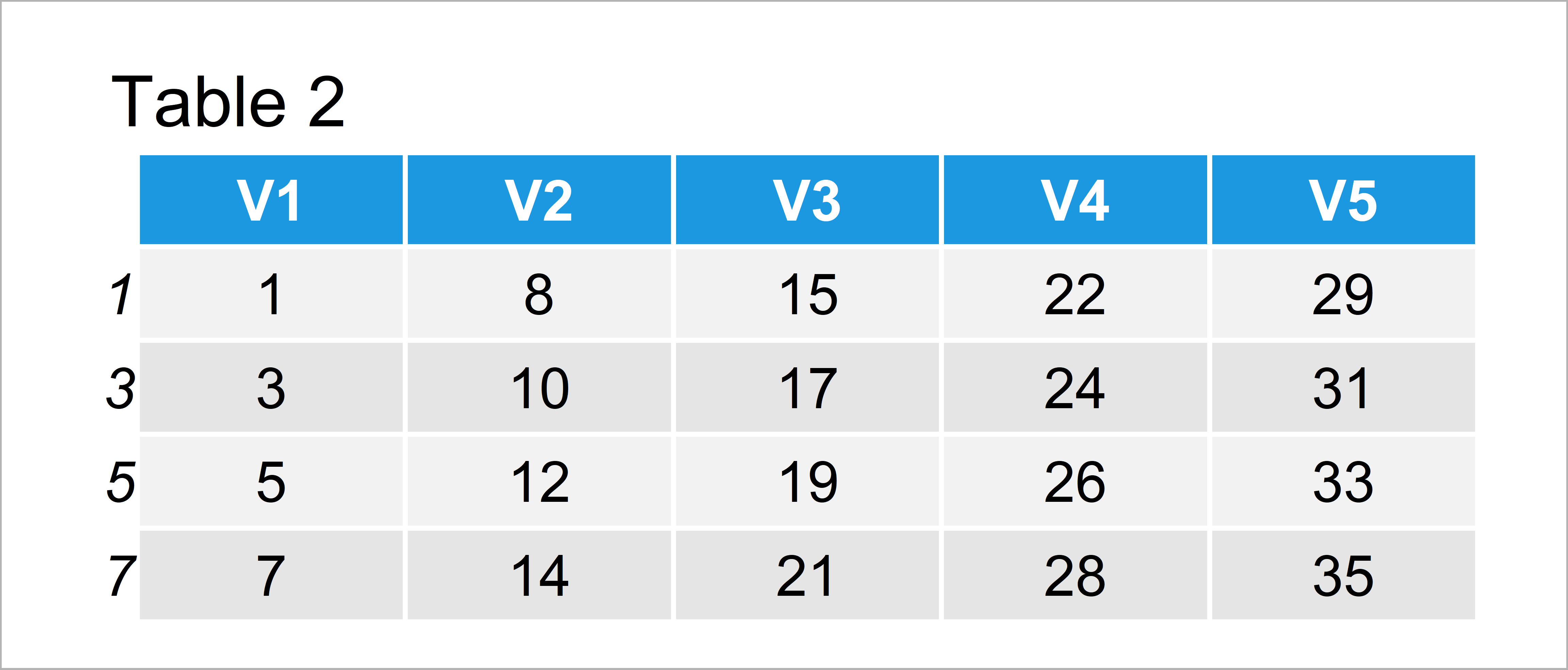By running the previous code we have created Table 2, i.e. a subset of our input data containing only the rows with an uneven index.

## Example 2: Extract Even Rows from Data Frame

In Example 2, I’ll illustrate how to retain only rows with an even index position.

For this task, we can use the dummy indicator that we have created in the previous example as shown below:

```data_row_even <- data[row_odd == 0, ]            # Subset even rows
data_row_even                                    # Print even rows```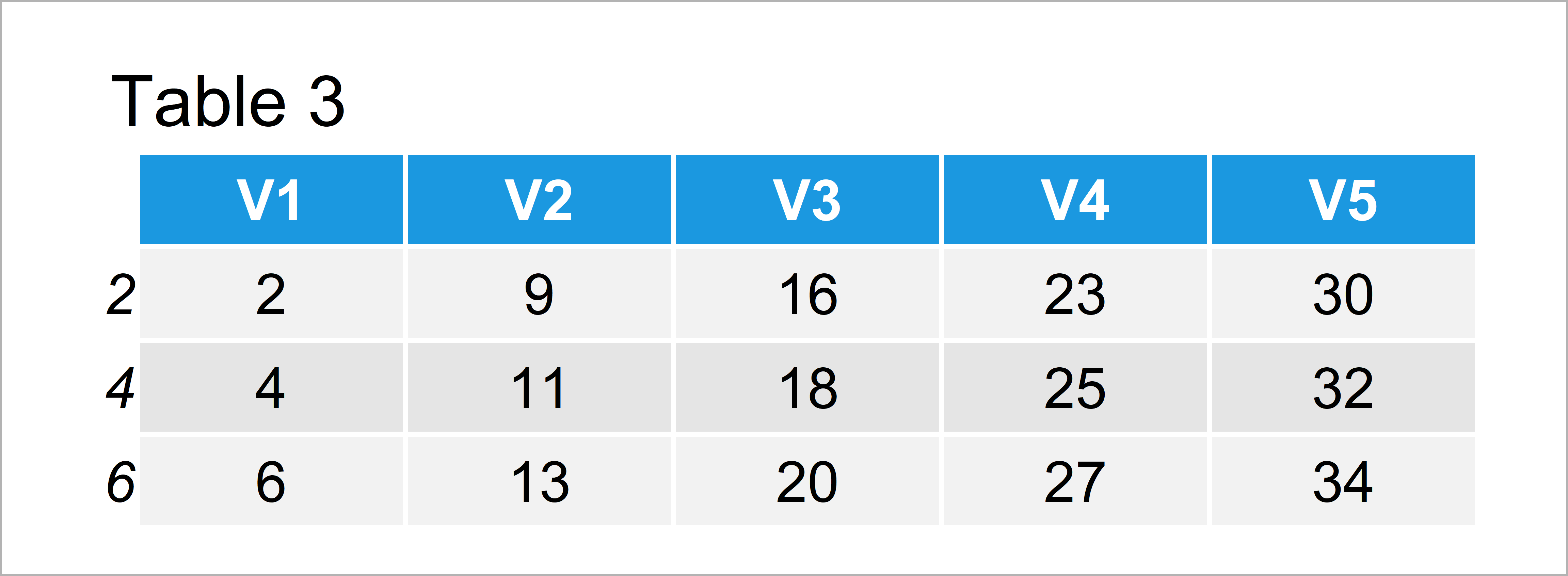The output of the previous syntax is shown in Table 3: A data frame consisting only of even row numbers.

## Example 3: Extract Odd Columns from Data Frame

In this example, I’ll explain how to keep only odd data frame variables in our data.

Similar to Example 1, we have to create a dummy indicator first(this time based on the ncol function):

```col_odd <- seq_len(ncol(data)) %% 2              # Create column indicator
col_odd                                          # Print column indicator
#  1 0 1 0 1```

Next, we can use this dummy to subset only odd columns:

```data_col_odd <- data[ , col_odd == 1]            # Subset odd columns
data_col_odd                                     # Print odd columns```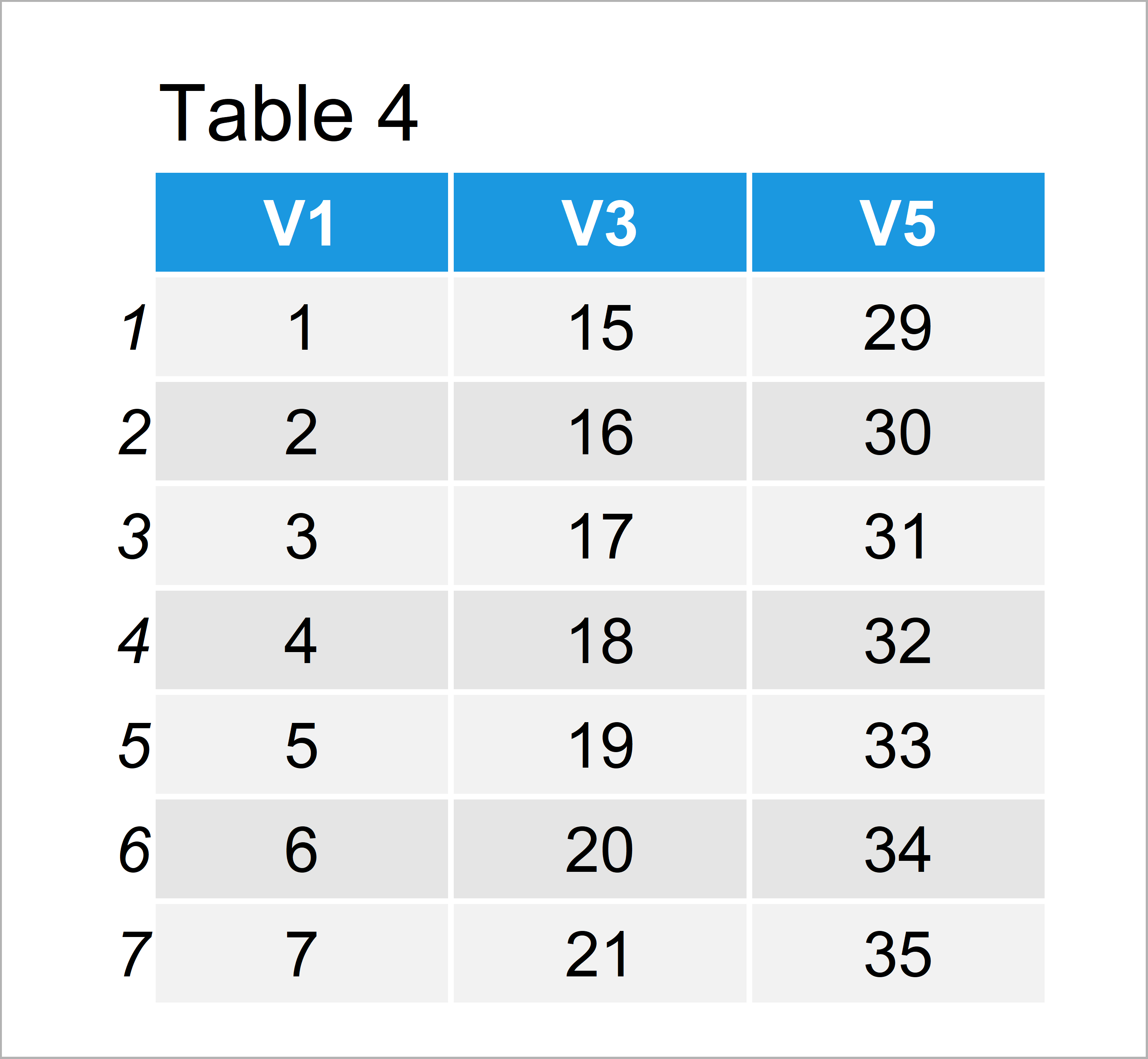After running the previous R programming syntax the data frame shown in Table 4 has been created. As you can see, we have removed all columns with even indices.

## Example 4: Extract Even Columns from Data Frame

Example 4 explains how to select all even columns of a data frame.

For this, we can use the dummy indicator of the previous example:

```data_col_even <- data[ , col_odd == 0]           # Subset even columns
data_col_even                                    # Print even columns```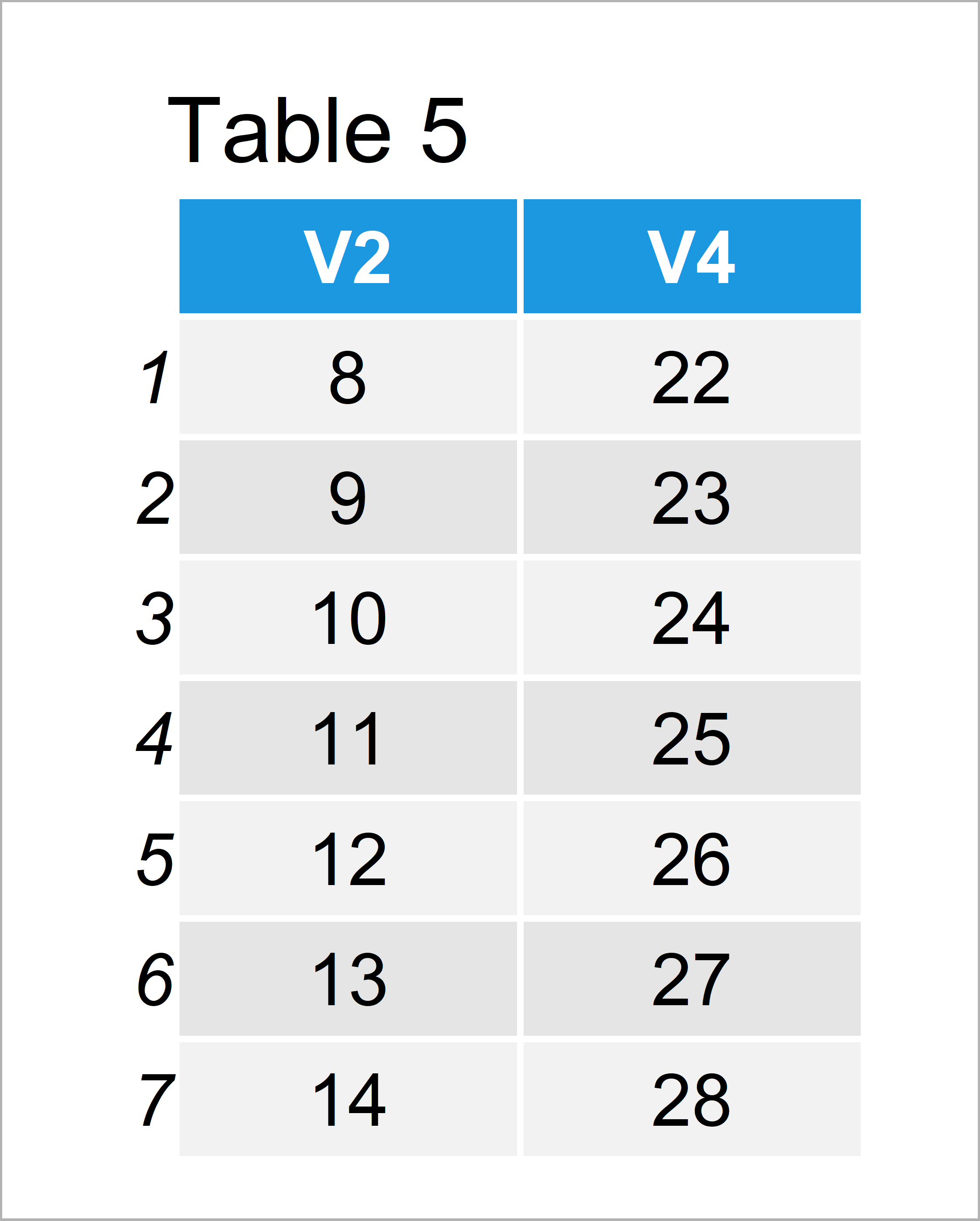As shown in Table 5, we have managed to construct another data frame using the previous R programming code. This data matrix contains only even columns.

## Video, Further Resources & Summary

I have recently released a video tutorial on my YouTube channel, which explains the R syntax of this article. You can find the video below:

Please accept YouTube cookies to play this video. By accepting you will be accessing content from YouTube, a service provided by an external third party.If you accept this notice, your choice will be saved and the page will refresh.

Besides that, you might want to read the related articles of this website.

In this R tutorial you have learned how to subset odd and even rows and columns. Let me know in the comments section below, if you have additional questions.

Subscribe to the Statistics Globe Newsletter

•Rusty Shackleford
August 31, 2022 3:14 pm

Incredibly useful, thanks for the nice write up.

•September 2, 2022 8:44 am